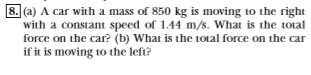8. (a) A car with a mass of 850 kg is moving to the rightwith a constant speed of 1.44 m/s. What is the totalforce on the car? (b) What is the total force on the carif it is moving to the left?

Questionhelp_outlineImage Transcriptionclose8. (a) A car with a mass of 850 kg is moving to the right with a constant speed of 1.44 m/s. What is the total force on the car? (b) What is the total force on the car if it is moving to the left? fullscreen
Step 1

(a)

Newton's second law states that acceleration of an object is directly proportional  to the net force acting on it and inversely proportional to its mass. If the acceleration of an object is zero, then the net force acting on it will be zero. Acceleration is the rate of change of velocity of an objcet. If the velocity of an object is constant, then the object will be having zero acceleration. Velocity is also a vector which has both magnitude and direction. This implies the acceleration will have a non zero value , either when the speed of the object changes or when its direction of motion changes. Here the car is moving with constant speed to the right. Neither the magnitude nor the direction of velocity changes so that the net acceleration and hence the total force on the car is zero.

Step 2

(b)

The car is moving with constant speed to the left. Neither the magnitude nor the direction of velocity ...

Want to see the full answer?

See Solution

Want to see this answer and more?

Our solutions are written by experts, many with advanced degrees, and available 24/7

See Solution
Tagged in

Physics